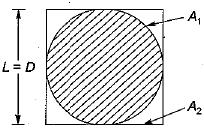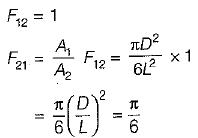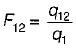# Radiation Heat Transfer - 2

## 10 Questions MCQ Test Topicwise Question Bank for GATE Mechanical Engineering | Radiation Heat Transfer - 2

Description
This mock test of Radiation Heat Transfer - 2 for Mechanical Engineering helps you for every Mechanical Engineering entrance exam. This contains 10 Multiple Choice Questions for Mechanical Engineering Radiation Heat Transfer - 2 (mcq) to study with solutions a complete question bank. The solved questions answers in this Radiation Heat Transfer - 2 quiz give you a good mix of easy questions and tough questions. Mechanical Engineering students definitely take this Radiation Heat Transfer - 2 exercise for a better result in the exam. You can find other Radiation Heat Transfer - 2 extra questions, long questions & short questions for Mechanical Engineering on EduRev as well by searching above.
QUESTION: 1

### What is the view factor F21 for sphere of diameter D inside a cubical box of length L = D?Solution:QUESTION: 2

Solution:
QUESTION: 3

### On a summer day, a scooter rider feels more comfortable while on move than while at a stop light because

Solution:

More heat is. lost by forced convection as the value of heat transfer coefficient is large because it depends on fluid properties, velocity of flow and surface condition.

QUESTION: 4

Thermal radiation extends over the range of

Solution:
QUESTION: 5

Fraction of radiative energy leaving one surface that strikes the other surface is called

Solution:

Shape factor represent the fraction of the energy emitted by one surface and received by the other.Where,
q1 = Total energy emitted by body 1
q12 = Energy emitted by (1) and received by (2)

QUESTION: 6

Shape factor can be termed a

Solution:
QUESTION: 7

Wave length for maximum power is given by

Solution:
QUESTION: 8

Stefan-Boltzmann’s law is applicable to

Solution:
QUESTION: 9

What is the basic equation of thermal radiation from which all other equations of radiation can be derived

Solution:
QUESTION: 10

Which one of the following is the correct representation of reciprocity theorem

Solution: